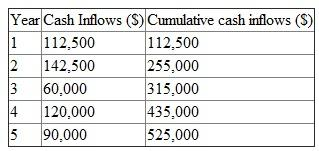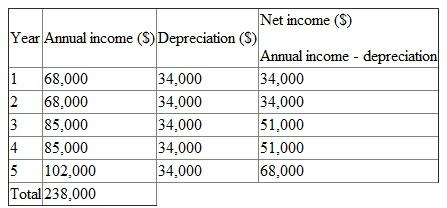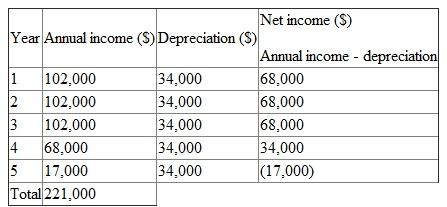## Quiz 19 : Capital InvestmentLooking for Accounting Homework Help?# Quiz 19 : Capital Investment

1. The payback period is the time required by the project to generate cash flows to cover its initial investment. For the even cash flows, it can be calculated by dividing initial investment with the annual cash flows. Hence, payback period for storage facility will be:Thus, payback period for storage facility is 3 years. 2. The payback period for uneven cash flows can be calculated by doing the fraction of the years generating cash inflows. In the case of car wash facility, the initial investment is \$360,000 and \$315,000 will be recovered in 3 years. The remaining \$45,000 of the initial investment will be recovered somewhere in 4 th year. Let us prepare the table of cumulative cash inflows:So, the payback period will be:Thus, the payback period of the car wash facility is 3.375 years. Hence, on the basis of payback period; storage facility should be accepted because in this project, the initial investment is recovering faster. 3. The payback period for laundry facility will be:Thus, payback period for laundry facility is 2.4 years. Now, on the basis of payback period; laundry facility should be accepted because in this project, the initial investment is recovering faster.

The process of making the capital investment decisions is known as capital budgeting. In capital budgeting basically two types of projects will be considered which are given below: • Independent projects • Mutually exclusive projects Independent projects are those projects whose acceptance or rejection does affect the cash flows of other projects, whereas the mutually exclusive projects are those projects whose acceptance precludes the acceptance of all other competing projects.

1. The net income of the project in given case when any other expense is not given is the annual income less depreciation expense. The annually depreciation can be calculated as:Now, the net income for each year will be:2. The accounting rate of return is based on the accounting method and hence considers profitability of the project. The accounting rate of return can be calculated by dividing average net income of the project with its initial investment. The accounting rate of return for the project will be:Thus, the accounting rate of return for the project is 0.28. 3. The annually depreciation of the second project will be same because it has same initial investment and salvage value. Now, the net income for each year will be:The accounting rate of return for the second project will be:Thus, the accounting rate of return for the second project is 0.26. Hence, on the basis of accounting rate of return first project should be selected as it has higher accounting rate of return. However, second project has higher cash inflows in initial years, thus will recover initial outlay faster.